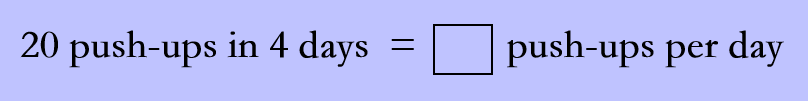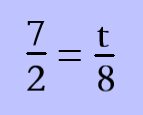# Understanding the Ratio and Rates

In this quiz, you will get to know about the concept of ratios and rates. You will find problems on equivalent ratios, unit rates, comparing the ratios, solving the proportion, and many more like these.

Start Quiz

What is the ratio of triangles to stars?4:2

2:4

4:6

Natasha’s town voted on a new law. There were 21 votes in favor of the law and 29 votes against the law. What is the ratio of the number of votes against the law to the number of votes in favor of the law?

21:29

29:21

29:50

Select all the ratios that are equivalent to 7:14.

1:2

3:9

4:8

Are these ratios equivalent?

7 hamburgers : 10 veggie burgers
14 hamburgers : 20 veggie burgers

Yes

No

Find the unit rate.4

6

5

The ratio of the side length of a square to the square's perimeter is always 1 to 4. Lewis drew a square with a perimeter of 36 inches.

What is the side length of the square Lewis drew?

6

9

12

Do the ratios 1/5 and 3/15 form a proportion?

yes

no

Solve the proportion.28

36

56

Jordan is using his birthday money to buy baseball cards. Danny's Downtown Shop sells boxes of 80 cards for \$23.20. The General Store sells boxes of 120 cards for \$28.80.

Which store offers the better deal?

Danny's Downtown Shop

The General Store

Quiz/Test Summary
Title: Understanding the Ratio and Rates
Questions: 9
Contributed by: